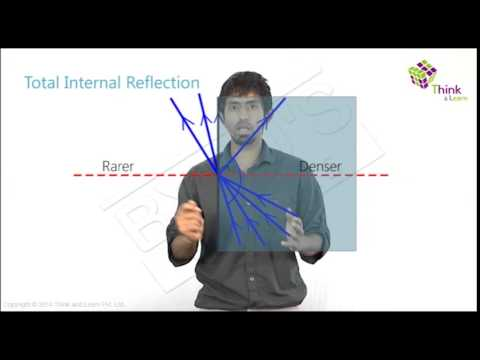# Relation Between Critical Angle And Refractive Index

In Optics, The angle of incidence to which the angle of refraction is 90° is called the critical angle. The ratio of velocities of a light ray in the air to the given medium is a refractive index. Thus, the relation between the critical angle and refractive index can be established as the Critical angle is inversely proportional to the refractive index.

## Critical Angle And Refractive Index

The relationship between critical angle and refractive index can be mathematically written as –

 $$\begin{array}{l}SinC=\frac{1}{\mu _{b}^{a}}\end{array}$$

Where,

C is the critical angle.

μ is the refractive index of the medium.

a and b represent two mediums in which light rays travel.

### Critical Angle And Refractive Index Formula

 Formula SI Unit Critical angle to Refractive index $$\begin{array}{l}SinC=\frac{1}{\mu _{b}^{a}}\end{array}$$ degree Refractive index to Critical angle $$\begin{array}{l}\mu _{b}^{a}=\frac{1}{sinC}\end{array}$$ No SI unit

## Critical angle and refractive index relation derivation

The relationship between critical angle and refractive index can be derived as –

### Consider a ray of light,

• Let the angle of incidence i be critical angle C
• Let the angle of refraction r=90º
• Refractive index of the rarer medium be μa
• Refractive index of the denser medium be μb

### Applying Snell’s Law

• $$\begin{array}{l}\frac{sin\;i}{sin\;r}=\frac{\mu _{a}}{\mu _{b}}\end{array}$$
• $$\begin{array}{l}\Rightarrow \mu _{b}sinC=\mu _{a}sin90^{0}\end{array}$$
• $$\begin{array}{l}\Rightarrow \frac{\mu _{b}}{\mu _{a}}=\frac{1}{sinC}\end{array}$$

Thus, we arrive at a formula expressing the critical angle and refractive index relation –

$$\begin{array}{l}\mu _{b}^{a}=\frac{1}{sinC}\end{array}$$

Hope you understood the relation and conversion between the Critical Angle and Refractive Index in Optics.

Physics Related Topics:

## Why do stars twinkle? Why do we see a delayed sunrise and sunset?Stay tuned to BYJU’S for more such interesting articles. Also, register to “BYJU’S-The Learning App” for loads of interactive, engaging physics-related videos and unlimited academic assistance.

Test Your Knowledge On Relation Between Critical Angle And Refractive Index!